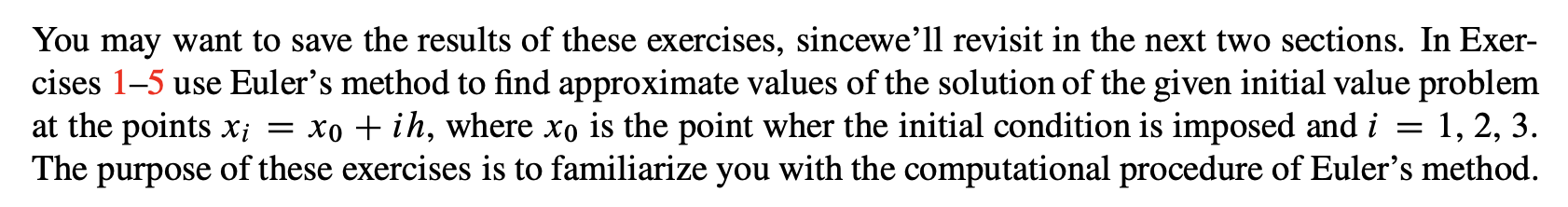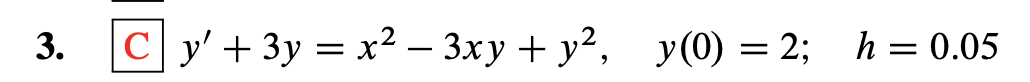# You may want to save the results of these exercises, sincewe’ll revisit in the next two sections. In Exer-cises 1-5 use Euler's method to find approximate values of the solution of the given initial value problemat the points x; = xo + ih, where xo is the point wher the initial condition is imposed and iThe purpose of these exercises is to familiarize you with the computational procedure of Euler's method.1, 2, 3. с у+ 3у %3Dх? — Зху + у?, у(0) %3D2;B h%3D0.053.

Question
1 views

Solution: y1 = 1.200000000; y2 =2.085625000; y3 = 3.079099746help_outlineImage TranscriptioncloseYou may want to save the results of these exercises, sincewe’ll revisit in the next two sections. In Exer- cises 1-5 use Euler's method to find approximate values of the solution of the given initial value problem at the points x; = xo + ih, where xo is the point wher the initial condition is imposed and i The purpose of these exercises is to familiarize you with the computational procedure of Euler's method. 1, 2, 3. fullscreenhelp_outlineImage Transcriptioncloseс у+ 3у %3Dх? — Зху + у?, у(0) %3D2;B h%3D0.05 3. fullscreen
check_circle

Step 1

Here, initial condition is given at x = 2.Therefore, start with x0 = 2.

Given equation is:

Step 2

First approximation at x1 = 0.05 is given by y1 which is calculated as:

Step 3

Now,

...

### Want to see the full answer?

See Solution

#### Want to see this answer and more?

Solutions are written by subject experts who are available 24/7. Questions are typically answered within 1 hour.*

See Solution
*Response times may vary by subject and question.
Tagged in

### Math